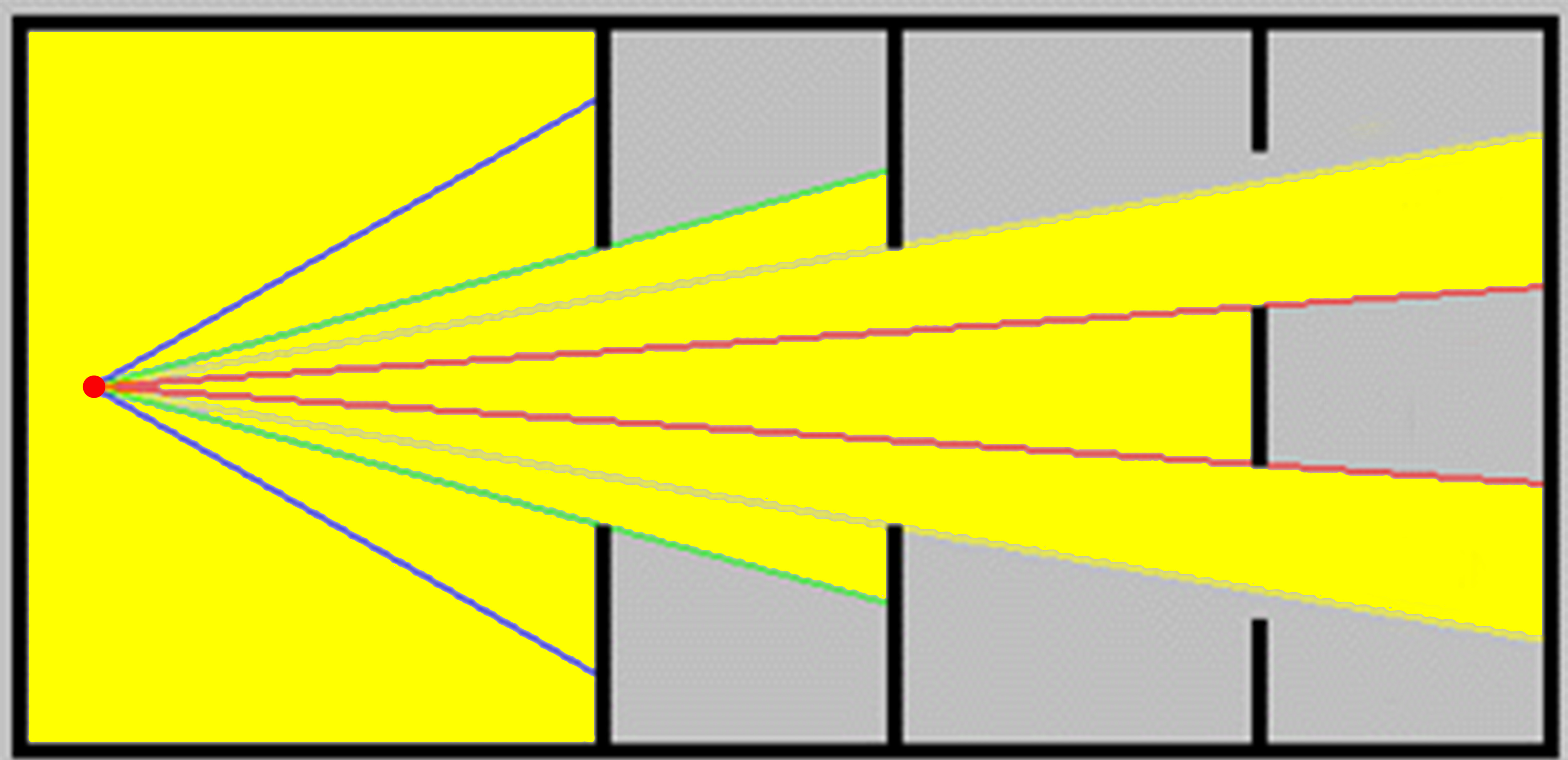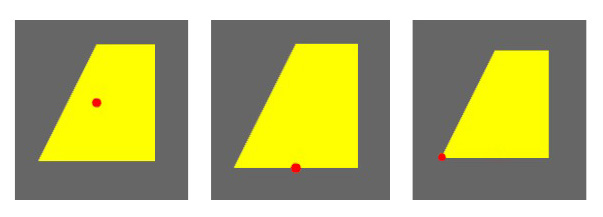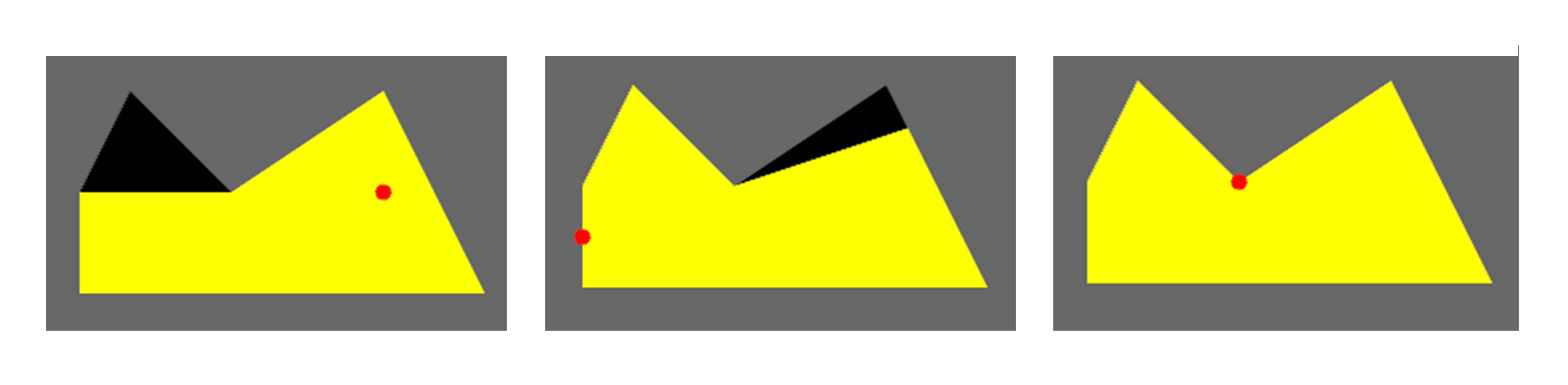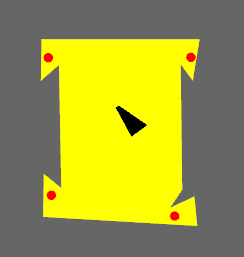# Computing Visibility Polygons## TL;DR

I discuss the gist and limitations of my implementation of Joe and Simpson’s visibility polygon algortihm, that is, an asymptotically optimal algorithm for computing the visibility polygon from a point inside of a simple polygon.

## The Problem

Recently, I took a course on computational geometry and got interested in the notion of visibility. Besides its applications in hidden surface removal (HSR) algorithms and exact robot motion planning, it received a great amount of attention through the art gallery problem. The first application I could think of when thinking about visibility were video games that incorporated a fog of war, that is, the computation of a player’s surroundings that is visible to him as he navigates a map. Unfortunately, the algorithm we will be talking about doesn’t work with obstacles, making it of limited use in games.

There are many different types of visibility problems, some deal with finding viewpoints to illuminate certain parts of the environment, while others deal with computing visible parts of the environment for a given viewpoint. Some of them consider a single viewpoint, some consider multiple viewpoints, and yet others consider different types of view elements than a point—the list goes on and on. In this post we will focus our attention on the following variant.

Problem Statement. Given a viewpoint $z$ inside of a simple polygon $P$ with $n$ vertices, we want to compute the visibility polygon $VP(P, z)$, which consists of all points in $P$ visible from the viewpoint $z$. We say that point $p$ is visible from point $q$ (and conversely, $q$ is visible from $p$) if and only if the line segment $\overline{pq}$ lies completely in $P$.

## Background

The visibility polygon from a single viewpoint $z$ can be computed naively in $\mathcal{O}(n^2)$ time. Simply cast a ray from $z$ towards every vertex of the polygon $P$ in, let’s say, counter-clockwise order. For each ray we iterate through all edges and store the closest (Euclidian distance to $z$) intersection as a vertex of the visibility polygon. Correctness follows from the observation that the visibility polygon’s boundary changes its shape only due to vertices of the polygon.

The above approach is simple yet computationally inefficient for large polygons. Fortunately, more efficient algorithms have been published. For example Asano’s $\mathcal{O}(nlogn)$ time sweeping algorithm and Joe and Simpson’s $\mathcal{O}(n)$ time algorithm (yes, those are the ones used by CGAL).

Quick Background of Joe and Simpson’s algorithm. Linear time algorithms have been shown to be optimal for computing the visibility polygon from a single viewpoint inside of a simple polygon. Such an algorithm was first proposed by ElGindy and Avis, it requires three stacks and is quite complicated. Then, a conceptually simpler algorithm requiring only one stack was proposed by Lee. Later, Joe and Simpson showed that both algorithms return wrong results for polygons that wind sufficiently, they published a correction of Lee’s algorithm. It is their algorithm that we’ll take a look at.

## The Algorithm

Instead of a futile attempt to try and capture the algorithm in more detail than Joe & Simpson did, I’ll present to you an overview that will be enough to understand the main idea. We’ll also take a closer look at parts of the algorithm for which there is no pseudocode in the paper—the pre- and post-processing.

The algorithm runs in $\mathcal{O}(n)$ time and space. It makes assumptions on the input which we establish in the preprocessing step—this produces a list of vertices $V = v_0, v_1, \cdots, v_n$ that represents the boundary of $P$ in a specific order, depending on the position of viewpoint $z$ as described in the paper (section two, first two paragraphs).

### Preprocessing

The preprocessing will shift the polygon such that the viewpoint $z$ becomes the new origin and it will rotate the polygon such that the closest vertex $v_0$ lies on the x-axis next to $z$—this ensures a line of sight between the first vertex in $V$ and our point of reference $z$ which makes the algorithm’s subsequent design simpler. We’ll make rotations simpler by working with coordinates in polar form.

	private static Pair<VsRep, Double> preprocess(CCWPolygon pol, Point2D z) {
// shift polygon such that z is origin
pol = pol.shiftToOrigin(z);

// determines the closest vertex to z
boolean zIsVertex = pol.vertices.contains(CommonUtils.origin2D);
PolarPoint2D v0 = getInitialVertex(pol, zIsVertex);

// converts the polygon's vertices from Cartesian to polar
List<PolarPoint2D> V = pol.vertices.stream().map(x -> new PolarPoint2D(x)).collect(Collectors.toList());

// adjusts list V such that v0 is at the beginning
placeV0First(V, v0);

// if z is on boundary then [v0, v1, ..., vk, z] -> [z, v0, v1, ..., vk]

// rotate all points of the shifted polygon clockwise such that v0 lies on the x axis
for (PolarPoint2D curr : V) {
if (!curr.isOrigin())
curr.rotateClockWise(v0.theta);
}
// return the preprocessed vertices and the angle of rotation
return new Pair(new VsRep(V, zIsVertex), v0.theta);
}


### Main Idea

Then the algorithm proceeds towards its three main procedures—Advance, Retard, and Scan—that handle the three different scenarios that can occur during the monotone scan of $V$—that is, of $P’s$ boundary. While iterating through $V$, a stack $S = s_0, s_1, \cdots, s_t$ of vertices is maintained—it represents a possible subset of vertices of the final visibility polygon. Note that vertices in $S$ are not necessarily vertices of the final visibility polygon. The three procedures are responsible for handling vertices while iterating through $V$ such that the final content of $S$ is all the information necessary for the postprocessing step to construct the final visibility polygon. Advance is responsible for pushing vertices from $V$ onto the stack $S$, retard for popping vertices from the $S$, and scan for skipping vertices in $V$ that have no business modifying $S$. Assume that $V$ is being scanned with $v_j, v_{j+1}$ as the current edge, and that $v_j$ is visible—for $v_{j+1}$ one of the following three cases can occur:

• $v_{j+1}$ is visible so that the newly discovered edge doesn’t obstruct previous vertices $\implies$ Advance is called—it pushes $v_{j+1}$ onto $S$.
• $v_{j+1}$ is visible and the newly discovered edge obstructs previous vertices $\implies$ Retard is called—it pops obstructed vertices from $S$ and pushes $v_{j+1}$.
• $v_{j+1}$ is invisible, it’s obstructed by previous vertices $\implies$ Scan is called—it doesn’t modify $S$ and keeps iterating through $V$ until it reaches a visible vertex.

The algorithm switches between the three procedures until $V$ is scanned completely—at this point $S$ contains all the necessary information to construct the final visibility polygon. The switching between procedures depends on the cumulative angular displacement of scanned vertices with respect to the viewpoint $z$—it is modified upon handling a new vertex from $V$, exactly how is described in the paper.

### Postprocessing

The preprocessing step modified the original input polygon $P$ in order to establish assumptions made by the rest of the algorithm—mainly that the first vertex $v_{0}$ that we process is visible which eliminates the need for subsequent special cases. The main algorithm therefore worked on a modified input polygon $P’$ and viewpoint $z’$ and therefore produced $VP(P’, z’)$ whose coordinates correspond to $P’$ and not to our original input polygon $P$.

The postprocessing converts $VP(P’, z’)$ to the corresponding visibility polygon in $P$ by taking $VP(P’, z’)$ and applying “inverse” operations to the ones applied to $P$ and $z$ during the preprocessing. Before shifting and rotating $VP(P’, z’)$ to obtain $VP(P, z)$ we have to reverse the stack’s content to establish counterclockwise order—we pushed vertices onto the stack while iterating in counterclockwise order, just popping them would give us $VP(P’, z’)$ in clockwise order.

	private static CCWPolygon postprocess(List<VertDispl> pre_s, VsRep V, Point2D z, double initAngle) {
if (V.zIsVertex)

// reverse order of stack to establish CCW order of final visibility polygon
Collections.reverse(pre_s);

// convert VertDispl to PolarPoint2D
List<PolarPoint2D> rotatedPol = pre_s.stream().map(v -> v.p).collect(Collectors.toList());

// rotates points back to original position before the rotation in preprocess()
for (PolarPoint2D curr : rotatedPol) {
curr.rotateClockWise(-initAngle);
}

// convert PolarPoint2D to Point2D
List<Point2D> shiftedPol = rotatedPol.stream().map(v -> v.toCartesian()).collect(Collectors.toList());

// shifts points back to their position before the shift in preprocess()
for (Point2D curr : shiftedPol)
curr.setLocation(curr.getX() + z.getX(), curr.getY() + z.getY());

return new CCWPolygon(shiftedPol);
}


Time and space complexity. Each vertex is scanned just once, at most two vertices are pushed onto the stack $S$ at each iteration, and popped vertices are never pushed again. This implies that $V$ and $S$ contain a linear number of vertices with respect to the input polygon’s size $n$. Hence, the algorithm runs in $\mathcal{O}(n)$ time and space.

What I left out. The degenerate cases and intricacies regarding the angular displacement and how this affects the switching between advance, scan, and retard with a detailed example on how exactly this algorithm avoids errors made by previously published algorithms is contained in the paper. It also contains the rationale that justifies the use of angular displacements and of course a proof of the algorithm’s correctness.

## Tests

I included tests of the pre- and post-processing steps, see TestPreprocessing.java and TestVisibilityPol.java for details. All of the following visualizations can be reproduced via the DrawVisibilityPolygons.java file.

Given the input polygon $P$ and a viewpoint $z$ I created tests for the following six scenarios. The polygon can be either convex or concave, for both types $z$ can be in $P$’s interior, or on an edge of $P$’s boundary, or on one of $P$’s vertices. The following figures are visualizations of those six scenarios.The algorithm can also be used to create the illusion of computing the visibility region from multiple viewpoints, that is, all points in $P$ visible from at least one of the viewpoints. I say illusion because the algorithm actually computes the visibility polygon for each of the viewpoints individually and unions them by drawing them onto the same plane. This points to a natural approach to actually compute the visibility region, we could use Joe and Simpson’s algorithm to compute the visibility polygons individually and union them with, say, Martinez et al.’s algorithm.## Usage

The VisibilityPolygon class can be used to compute the visibility polygon from a point inside of a simple polygon (given as n vertices in counterclockwise order) in O(n) time and space. Here is an example:

    // initialize polygon vertices in CCW order
List<Point2D> vertices = new ArrayList<>();

// initialize polygon
CCWPolygon pol = new CCWPolygon(vertices);

// initialize viewpoint
Point2D z = new Point2D.Double(4, 4);

// VP contains the visibility polygon from z in pol in CCW order.
CCWPolygon VP = VisibilityPolygon.computeVisPol(pol, z);


## Robustness Issues

Substituting floating-point arithmetic for the real arithmetic assumed in the paper doesn’t go unpunished. My implementation will fail for certain inputs due to round-off errors cause by the inherent limitations of floating-point arithmetic. A straightforward solution would be to make use of some library that allows for arbitrary-precision arithmetic such as Apfloat or JScience.

Another approach for obtaining a less robust but presumably more efficient implementation would be to modify the predicates and experimentally assess the improvement in robustness. In order to implement the above algorithm in a robust manner, it is necessary to robustly implement the predicates upon which it relies. The algorithm repeatedly runs a two dimensional orientation test to determine whether a point lies to the left of, to the right of, or on a line defined by two other points. It also computes intersections between lines and segments, half-lines and segments, and between two segments. We will see that all the predicates can be reduced to orientation tests.

For the orientation test we use the determinant approach—it’s fast and immediately applicable to double precision floating-point inputs.

The orientation test is performed by evaluating the sign of $orientation(A, B, C)$:

\begin{equation} orientation(A, B, C) = \begin{vmatrix} a_x & a_y & 1 \\ b_x & b_y & 1 \
c_x & c_y & 1 \end{vmatrix} = \begin{vmatrix} a_x - c_x & a_y - c_y \\ b_x - c_x & b_y - c_y \end{vmatrix} \end{equation}

If $orientation(A, B, C)$ is less than 0 then $C$ lies to the right of the line that goes through $A$ and $B$, if greater than 0 then $C$ is to the left of, and if equal to 0 then $C$ lies on the line.

Next we’ll take a look at the connection between the orientation test and other geometric predicates that my implementation uses. The problem of testing whether a line and a segment intersect can be reduced to two orientation tests. To test whether a line $l$ and a line segment $ls$ intersect we test whether an endpoint of $ls$ lies on $l$ or whether the interior of $ls$ intersects $l$. For the former we simply test whether endpoints of $ls$ lie on $l$ with the line equation in point-slope form. Testing whether the interior of $ls$ intersects $l$ is equivalent to testing whether the endpoints of $ls$ lie on opposite sides of $l$—can be determined with two orientation tests. The other tests can be reduced similarly—they have more special cases, see the provided implementation for details. The fact that all predicates are reducible to orientation tests makes me believe that implementing the orientation test in a robust manner could significantly improve the algorithm’s robustness.

At this point I’ll quote Schirra’s advice that is directly applicable to our problem:

The straightforward approach to implement geometric algorithms reliably is to use exact rational arithmetic instead of inherently imprecise floating-point arithmetic. Unfortunately, this slows down the code by orders of magnitude. As suggested by the exact geometric computation paradigm a better approach is to combine exact rational arithmetic with floating-point filters, e.g. interval arithmetic, in order to save most of the efficiency of floating-point arithmetic for nondegenerate cases. This approach is implemented in the exact geometry kernels of CGAL and LEDA. The use of adaptive predicates `a la Shewchuck is highly recommended.

Therefore, the algorithm’s robustness issues could be partially resolved by replacing the current naive implementation of the orientation test by Shewchuk’s adaptive approach. It would be interesting to compare the impact on robustness and running time when substituting the current orientation test for Shewchuk’s more robust but presumably slower approach. Note that my implementation would still fail after the substitution because of round-off errors—even if all orientation tests are performed flawlessly—due to comparisons between doubles that are sprinkled all over the code.

### Summary

In this post we discussed the gist behind Joe and Simpson’s algorithm for computing the visibility polygon from a viewpoint inside of a simple polygon and concluded by taking a look at the robustness issues of my implementation. We saw how to establish assumptions about the input that were made in the paper—the preprocessing step. Then, we discussed the main idea behind the three routines—Advance, Retard and Scan—and in what manner they modify the stack. We took a look at how the final visibility polygon is constructed using the stack’s content left by the three subroutines after the algorithm finished iterating through the polygon’s boundary—the postprocessing step. We concluded with a discussion on robustness issues where we mentioned an approach to resolve them—arbitrary-precision arithmetic —and an idea for future work that makes use of adaptive predicates.